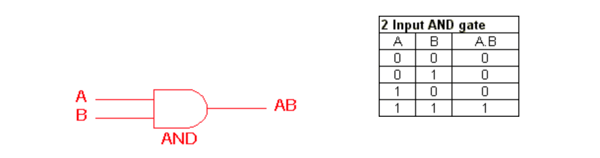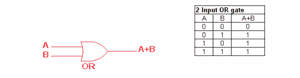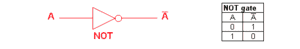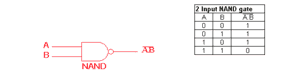Trusted answers to developer questions
Trusted Answers to Developer Questions

Related Tags

logic
gates
and
or
not

# Logic gatesEducative Answers Team

Grokking Modern System Design Interview for Engineers & Managers

Ace your System Design Interview and take your career to the next level. Learn to handle the design of applications like Netflix, Quora, Facebook, Uber, and many more in a 45-min interview. Learn the RESHADED framework for architecting web-scale applications by determining requirements, constraints, and assumptions before diving into a step-by-step design process.

Logic gates are the fundamental building blocks of circuits. Circuits are used to perform operations in a computer system. They are mainly used in the arithmetic logic unit known as ALU.

There are 5 main logic gates.

• AND
• OR
• NOT
• NOR
• NAND

These logic gates take inputs and give a single output.

## AND gate

The AND gate gives $1$ as an output when all the inputs are $1$. If there are any $0$'s in the input, the AND gate gives $0$ as an output. Think of it as multiplication between $0$ and $1$.## OR gate

The OR gate gives $1$ as an output when at least one of the inputs is $1$. If all inputs are $0$, the output is $0$. Think of it as addition between $0$ and $1$.## NOT gate

NOT is a simple gate that takes in a single input and reverses its value. Whatever the input, this gate outputs the opposite (i.e., if $1$ is inputted, it gives $0$ as the output).## NOR gate

The NOR gate is opposite to the OR gate. It gives $1$ as an output whenever all inputs are $0$ (i.e., $1$ is not an input).## NAND gate

The NAND gate gives $1$ as an output when there is a single $0$ as an input. It gives $0$ as an output whenever all inputs are $1$. NAND is opposite of AND.RELATED TAGS

logic
gates
and
or
not